# Clicker Heroes

 93 7 42M•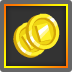complete
•complete
•complete
•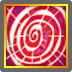complete
•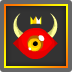complete
•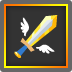complete
•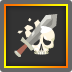complete
 Plays 41,677,474 Ratings 34,116 Favorites 36,443 Published Sep 22, 2014

## Description

Kill monsters, collect gold, upgrade heroes, use skills, find treasure, kill bosses, and explore new worlds in this epic adventure!

## Controls

Click on the monsters.

Legend of Large Numbers
1K = 1,000 = One Thousand
1M = 1,000K = One Million
1B = 1,000M = One Billion
1T = 1,000B = One Trillion
1q = 1,000T = One Quadrillion
1Q = 1,000q = One Quintillion
1s = 1,000Q = One Sextillion
1S = 1,000s = One Septillion
1O = 1,000S = One Octillion
1N = 1,000O = One Nonillion
1d = 1,000N = One Decillion
1U = 1,000d = One Undecillion
1D = 1,000U = One Duodecillion
1! = 1,000D = One Tredecillion
1@ = 1,000! = One Quattuordecillion
1# = 1,000@ = One Quindecillion
1\$ = 1,000# = One Sexdecillion
1% = 1,000\$ = One Septendecillion
1^ = 1,000% = One Octodecillion
1& = 1,000^ = One Novemdecillion
1* = 1,000& = One Vigintillion
A lot > 1,000* < A lot

## November 27, 2017

Hey Heroes, This is an early warning for patch 1.10. It will be arriving in several days, and will have a lot of changes! If you've transcended ever, the game will require you to transcend upon loading into the 1.10 update!

## Popular Articles